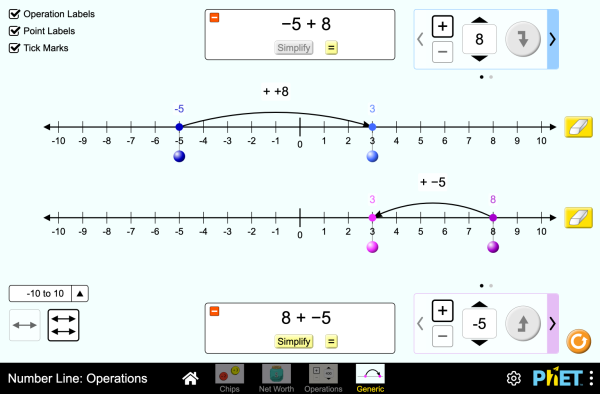# Number Line: Operations

هذه التقنية لم تتم ترجمتها لهذه اللغة. غير أنه لا يزال بإمكانك الدخول إلى النسخة الإنجليزية أدناه.تحميلEmbed close Embed a running copy of this simulation Use this HTML to embed a running copy of this simulation. You can change the width and height of the embedded simulation by changing the "width" and "height" attributes in the HTML. Embed an image that will launch the simulation when clicked
Click to Run
Use this HTML code to display a screenshot with the words "Click to Run". Operations Number Line Integers PhET is supported byand educators like you.

• Operations
• Number Line
• Integers

### Description

Explore operations with integers using an authentic context of net worth, then generalize with a number line. Experiment with adding and subtracting both positives and negatives, and make predictions about whether the total will be positive or negative.

### أهداف التعلم

• Represent addition and subtraction of integers on a horizontal number line
• Reason about addition and subtraction of integers in terms of number-locations
• Use logical necessity to reason that addition has the opposite effect as subtraction or that adding (or subtracting) a negative integer has the opposite effect as adding (or subtracting) a positive integer.
• Recognize and generate equivalence classes of integer sums and differences
• Show that a number and its additive inverse (opposite) have a sum of 0
• Using a net worth context, describe situations that have a positive sum or difference, negative sum or difference, and zero sum or difference
• View adding a negative as equivalent to subtracting a positive, and explain why this relationship makes sense in contexts such as net worth.
• View subtracting a negative as equivalent to adding a positive, and explain why this relationship makes sense in contexts such as net worth.
• Apply a number line model for addition and subtraction to new contexts.

### Standards Alignment

#### Common Core - Math

7.NS.A.1
Apply and extend previous understandings of addition and subtraction to add and subtract rational numbers; represent addition and subtraction on a horizontal or vertical number line diagram.
7.NS.A.1a
Describe situations in which opposite quantities combine to make 0. For example, a hydrogen atom has 0 charge because its two constituents are oppositely charged.
7.NS.A.1b
Understand p + q as the number located a distance |q| from p, in the positive or negative direction depending on whether q is positive or negative. Show that a number and its opposite have a sum of 0 (are additive inverses). Interpret sums of rational numbers by describing real-world contexts.
7.NS.A.1c
Understand subtraction of rational numbers as adding the additive inverse, p - q = p + (-q). Show that the distance between two rational numbers on the number line is the absolute value of their difference, and apply this principle in real-world contexts.
7.NS.A.1d
Apply properties of operations as strategies to add and subtract rational numbers.
Version 1.0.1

### Teacher TipsThe دليل المعلم (pdf) يشتمل على نصائح للمعلمين تم وضعها بواسطة فريق موقع فيت. ( PDF ).

### Teacher-Submitted Activities

Integer Addition and Subtraction Assessment Gillian Walker المرحلة المتوسطة
المرحلة الثانوية
واجب منزلي
Remote
معمل
الرياضيات
الإسبانية All españolRecta Numérica: Operacionesالإسبانية<br/>(البيرو) All español (Perú)Recta numérica: Operaciones
الألمانية All DeutschZahlenstrahl: Operationen
الإيطالية All italianoRetta dei numeri: operazioni
الباسك All EuskaraZenbaki-lerroa: eragiketak
البرتغالية البرازيلية All português (Brasil)Reta Numérica: Operações
البوسنية All BosanskiBrojevna prava: Operacije
البولندية All polskiOś liczbowa: działania
التركية All TürkçeSayı Doğrusu: İşlemler
الدنمركية All DanskTallinje: Beregninger
السلوفاكية All SlovenčinaČíselná os: Operácie
الصربية All СрпскиБројевна права: Операције
الصينية- التقليدية All 中文 (台灣)Number Line: Operations_數線：數線的運算
الفرنسية All françaisDroite numérique: opérations
الفيتنامية All Tiếng ViệtĐường thẳng số: Các phép toán
الكرواتية All hrvatskiBrojevna crta: Računske operacije
اللاتفية All LatviešuSkaitļu ass: Darbības
الموارية All MaoriRārangi Tau: Paheko
الهنغارية All magyarSzámegyenes - műveletek
الهولندية All NederlandsGetallijn: Bewerkingen
اليونانية All ΕλληνικάΑριθμογραμμή: πράξεις

HTML5 sims can run on iPads and Chromebooks, as well as PC, Mac, and Linux systems on modern web browsers. If you are experiencing issues using an HTML5 sim on a supported platform, please disable any browser extensions.

iOS 12+ Safari

Android:
Not officially supported. If you are using the HTML5 sims on Android, we recommend using the latest version of Google Chrome.

Chromebook:
The HTML5 and Flash PhET sims are supported on all Chromebooks.
Chromebook compatible sims

Windows Systems: